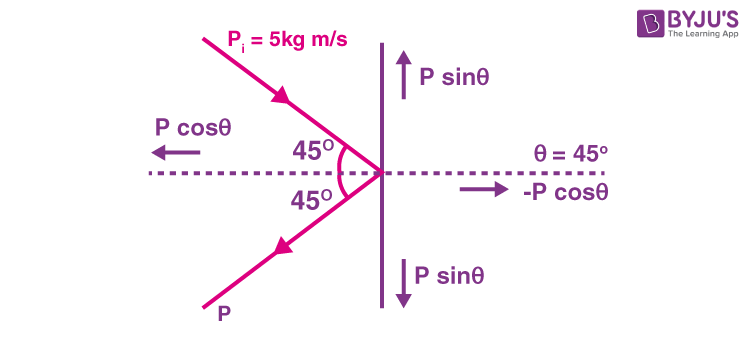# A ball moving with a momentum of 5kgm/s strikes against a wall at angle of 45 degree and is deflected at the same angle. Calculate the change in momentum.

Given

P = 5 kg/s

θ = 450

From the figure, there is no change in perpendicular component and cancel each other

The initial momentum along x axis,

Pi = P cos θ

The final momentum along x axis,

Pf = -P cos θ

Hence,

Change in momentum,

ΔP = Pf – Pi

ΔP = -P cos θ – P cos θ

ΔP = -2P cos θ

Substituting the value of P and θ, we get,

ΔP = -2 x 5 cos 450

We get,

ΔP = -7.071 kgm/s

-is the change in momentum(0)(0)